Aliasing (Aliasing Noise)

The sampling theorem demands that the sampling frequency be at least two times the highest frequency contained in the input signal. The frequency which is 1/2 times the sampling frequency is referred to as the Nyquist frequency. If the input signal contains a component which is higher than the Nyquist frequency, aliasing occurs.

Amplitude Probability Density Function

The amplitude probability density function obtains the probability that a varying signal exists at a specific amplitude value. The horizontal axis denotes the amplitude (V) and the vertical axis is normalized from 0 to 1. With this software, the amplitude is decomposed to 1/512 times the voltage range. The amplitude probability density function makes it possible to analyze how the input signal varies near what portion of the amplitude, and can be used for the PASS/FAIL test by shape.

Amplitude Probability Distributions Function

This function gives the probability that the instantaneous amplitude value of a varying time-axis signal is equal to or less than a certain level. The amplitude probability distribution function can be obtained by integrating the amplitude probability density function.

Analysis Data Length

The number of data points for performing the FFT operation which is the n-th power of 2.

An analysis data length of 64, 128, 256, 512, 1,024, 2,048, and 4,096 points is subject to FFT operation to obtain the frequency data of 25, 50, 100, 200, 400, 800 and 1,600 points, respectively.

Frequency resolution HREF_FRQ_RESOL depends on the number of points for FFT operation.

Autocorrelation Function

The auto-correlation function is a function of delay amount τ when waveform x (t) and x (t+τ) which is shifted from x (t) by time τ are used. It is defined by the following expression: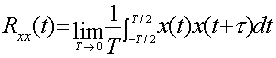The auto-correlation function is effective for investigating the period of a waveform. The auto-correlation function gives the maximum value when τ=0, i.e., resulting in the product of itself. If the waveform is periodic, the auto-correlation function gives a peak in the same period. If an irregular signal changes slowly, it gives a large value when τ is large; if the signal changes rapidly, it gives a large value when τ is small. The auto-correction function allows you to use τ as a temporal reference for variation.

With the FFT analyzer, the auto-correlation function is obtained by the inverse Fourier transform of the power spectrum.

Averaging

Types of averaging

• Summation averaging, normalize summation averaging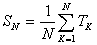N summation averages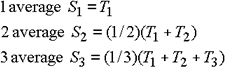• Exponential averaging

In exponential averaging, not the number of averages but the numerical value for weighting of the latest data is set. This number is equivalent to the time constant of the analog RC filter.

When N=4,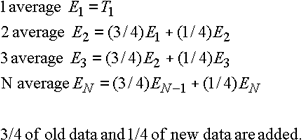• Peak hold

Peak hold of the power spectrum is performed. The maximum value for each frequency line from the peak hold start to pause is held (memorized).

In conjunction with this peak hold, the max. overall function is provided. This function is assumed to be the peak hold function of the overall value and the function to memorize the power spectrum when the overall value is the maximum value. In this peak hold mode, there is no number-of-average setting. Therefore, in the averaging mode, start and pause (stop) operations are required. Even if the number of averages has already been set, peak hold is not related. The number of CRT executions increases also when this peak hold is executed. This shows the number of FFT operations.

 Caution In the peak hold mode, operation between channels etc. cannot be performed and therefore it is not possible to display the average result of the following functions:Cross spectrum, transfer function, coherence function, coherent output power, and impulse response

• Subtraction averaging

Subtraction averaging is a function which subtracts the power spectrum from the power spectrum after summation averaging.

[Expressions]

Example: Number of subtraction averages N=20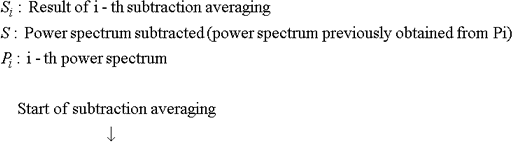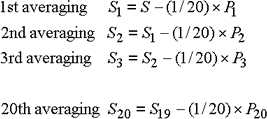• Sweep averaging

A sine signal is used to sweep from low frequency to high frequency, and FFT operation is performed according to the signal.

In sweep averaging, the maximum spectrum (one line) on the master channel side is detected for each sampling and calculation is performed only for the one line, and only the line is updated.

Note that, if the sweep speed of the external sweep signal is higher than the operation processing speed, unobtainable (missing) spectrum lines occur.

Domains and types for which averaging is possible

• Time domain averaging

Summation averaging, exponential averaging

 Caution In averaging in the time domain, synchronous summation using the trigger function is performed. Synchronous summation has an advantage that the analysis signal synchronized with the trigger signal contained in the input is separated from random noise. In averaging in the time domain, the phase information is also included and therefore it is necessary to give the sampling timing. Although averaging in the time domain is performed without using the trigger function (free run), the phase becomes random and then averaging becomes meaningless. The trigger function is used invariably.
• Frequency domain averaging

Summation averaging, exponential average, peak hold, subtraction averaging, sweep averaging

• Amplitude domain

Summation averaging

Bode Diagram

The Bode diagram is a diagram which represents the frequency characteristics consisting of a combination of gain characteristic and phase characteristic of frequency response function H (f). The vertical axis of the gain is assigned the unit of decibel (dB), based on 20 log (10) H (f), and the phase is expressed in degree or radian.

The Bode diagram makes it easier to read out the gain and phase margins used to determine the stability of control systems, which are displayed in the logarithmic scale with dBVrms.